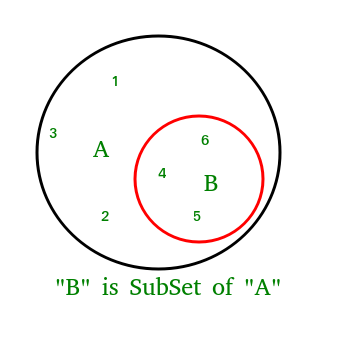Open in App
Not now

# Python Set issubset() Method

• Difficulty Level : Basic
• Last Updated : 25 Aug, 2022

Python set issubset() method returns True if all elements of a set A are present in another set B which is passed as an argument, and returns False if all elements are not present in Python.

## Python Set issubset() Method Syntax:

Syntax: set_obj.issubset(other_set)

Parameter:

• other_set: any other set to compare with.

Return: bool

## Python3

 `s1 ``=` `{``1``, ``2``, ``3``, ``4``, ``5``}``s2 ``=` `{``4``, ``5``}``# Output True, since, s2 elements in s1``print``(s2.issubset(s1))`

Output:

`True`Python set issubset() method

## Python

 `A ``=` `{``4``, ``1``, ``3``, ``5``}``B ``=` `{``6``, ``0``, ``4``, ``1``, ``5``, ``0``, ``3``, ``5``}`` ` `# Returns True``print``(A.issubset(B))`` ` `# Returns False``# B is not subset of A``print``(B.issubset(A))`

Output:

```True
False```

## Python

 `# Another Python program to demonstrate working``# of issubset().``A ``=` `{``1``, ``2``, ``3``}``B ``=` `{``1``, ``2``, ``3``, ``4``, ``5``}``C ``=` `{``1``, ``2``, ``4``, ``5``}`` ` `# Returns True``print``(A.issubset(B))`` ` `# Returns False``# B is not subset of A``print``(B.issubset(A))`` ` `# Returns False``print``(A.issubset(C))`` ` `# Returns True``print``(C.issubset(B))`

Output:

```True
False
False
True```

My Personal Notes arrow_drop_up